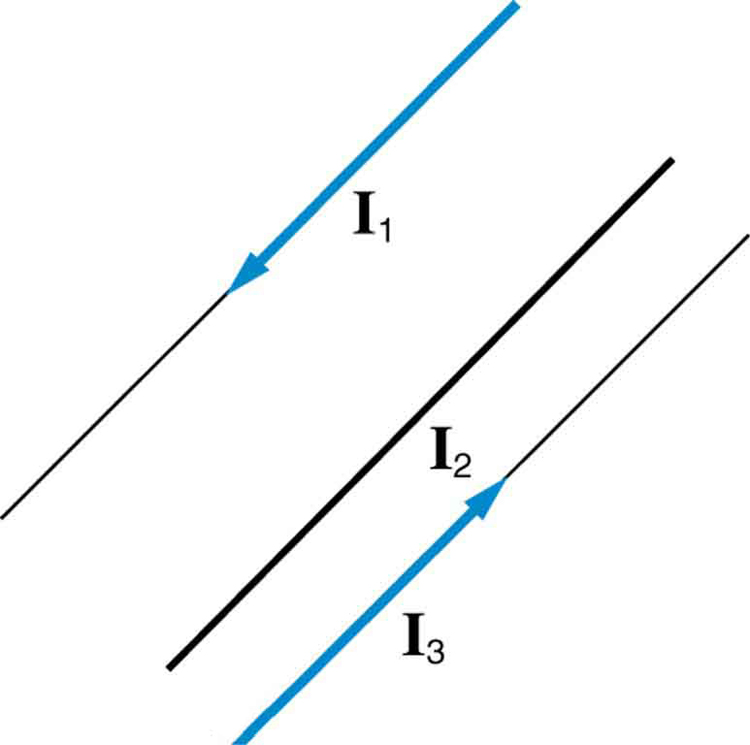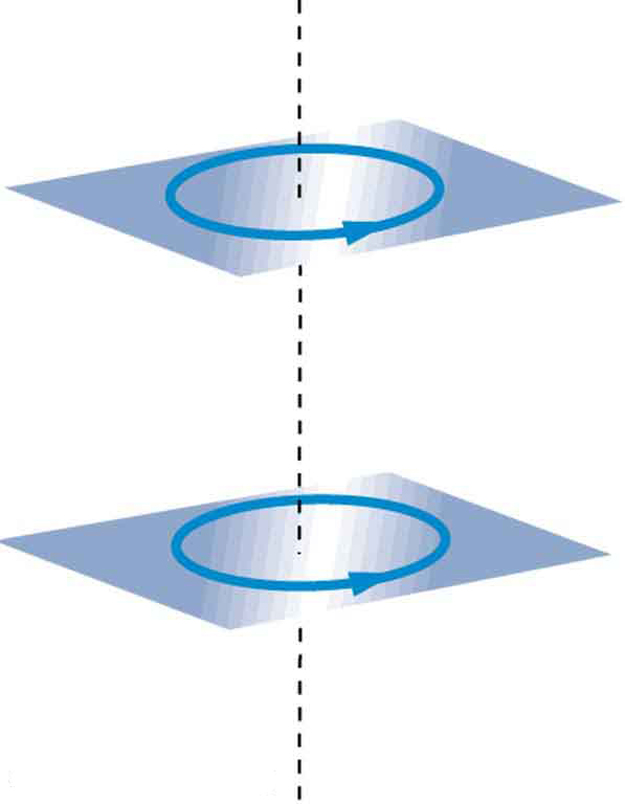# 22.10 Magnetic force between two parallel conductors  (Page 2/2)

 Page 2 / 2

This force is responsible for the pinch effect in electric arcs and plasmas. The force exists whether the currents are in wires or not. In an electric arc, where currents are moving parallel to one another, there is an attraction that squeezes currents into a smaller tube. In large circuit breakers, like those used in neighborhood power distribution systems, the pinch effect can concentrate an arc between plates of a switch trying to break a large current, burn holes, and even ignite the equipment. Another example of the pinch effect is found in the solar plasma, where jets of ionized material, such as solar flares, are shaped by magnetic forces.

The operational definition of the ampere is based on the force between current-carrying wires. Note that for parallel wires separated by 1 meter with each carrying 1 ampere, the force per meter is

$\frac{F}{l}=\frac{\left(4\pi ×{\text{10}}^{-7}\phantom{\rule{0.25em}{0ex}}T\cdot \text{m/A}\right){\left(1 A\right)}^{2}}{\left(2\pi \right)\left(1 m\right)}=2×{\text{10}}^{-7}\phantom{\rule{0.25em}{0ex}}\text{N/m.}$

Since ${\mu }_{0}$ is exactly $4\pi ×{\text{10}}^{-7}\phantom{\rule{0.25em}{0ex}}T\cdot \text{m/A}$ by definition, and because $\text{1 T}=\text{1 N/}\left(A\cdot m\right)$ , the force per meter is exactly $2×{\text{10}}^{-7}\phantom{\rule{0.25em}{0ex}}\text{N/m}$ . This is the basis of the operational definition of the ampere.

## The ampere

The official definition of the ampere is:

One ampere of current through each of two parallel conductors of infinite length, separated by one meter in empty space free of other magnetic fields, causes a force of exactly $2×{\text{10}}^{-7}\phantom{\rule{0.25em}{0ex}}\text{N/m}$ on each conductor.

Infinite-length straight wires are impractical and so, in practice, a current balance is constructed with coils of wire separated by a few centimeters. Force is measured to determine current. This also provides us with a method for measuring the coulomb. We measure the charge that flows for a current of one ampere in one second. That is, $1 C=1 A\cdot s$ . For both the ampere and the coulomb, the method of measuring force between conductors is the most accurate in practice.

## Test prep for ap courses

Two parallel wires carry equal currents in the same direction and are separated by a small distance. What is the direction of the magnetic force exerted by the two wires on each other?

1. No force since the wires are parallel.
2. No force since the currents are in the same direction.
3. The force is attractive.
4. The force is repulsive.

(c)

A wire along the y -axis carries current in the + y -direction. An experimenter would like to arrange a second wire parallel to the first wire and crossing the x -axis at the coordinate so that the total magnetic field at the coordinate is zero. In what direction must the current flow in the second wire, assuming it is equal in magnitude to the current in the first wire? Explain.

## Section summary

• The force between two parallel currents ${I}_{1}$ and ${I}_{2}$ , separated by a distance $r$ , has a magnitude per unit length given by
$\frac{F}{l}=\frac{{\mu }_{0}{I}_{1}{I}_{2}}{2\mathrm{\pi r}}.$
• The force is attractive if the currents are in the same direction, repulsive if they are in opposite directions.

## Conceptual questions

Is the force attractive or repulsive between the hot and neutral lines hung from power poles? Why?

If you have three parallel wires in the same plane, as in [link] , with currents in the outer two running in opposite directions, is it possible for the middle wire to be repelled by both? Attracted by both? Explain.Three parallel coplanar wires with currents in the outer two in opposite directions.

Suppose two long straight wires run perpendicular to one another without touching. Does one exert a net force on the other? If so, what is its direction? Does one exert a net torque on the other? If so, what is its direction? Justify your responses by using the right hand rules.

Use the right hand rules to show that the force between the two loops in [link] is attractive if the currents are in the same direction and repulsive if they are in opposite directions. Is this consistent with like poles of the loops repelling and unlike poles of the loops attracting? Draw sketches to justify your answers.Two loops of wire carrying currents can exert forces and torques on one another.

If one of the loops in [link] is tilted slightly relative to the other and their currents are in the same direction, what are the directions of the torques they exert on each other? Does this imply that the poles of the bar magnet-like fields they create will line up with each other if the loops are allowed to rotate?

Electric field lines can be shielded by the Faraday cage effect. Can we have magnetic shielding? Can we have gravitational shielding?

## Problems&Exercises

(a) The hot and neutral wires supplying DC power to a light-rail commuter train carry 800 A and are separated by 75.0 cm. What is the magnitude and direction of the force between 50.0 m of these wires? (b) Discuss the practical consequences of this force, if any.

(a) 8.53 N, repulsive

(b) This forceis repulsiveand thereforethere isnever arisk thatthe twowires willtouch andshort circuit.

The force per meter between the two wires of a jumper cable being used to start a stalled car is 0.225 N/m. (a) What is the current in the wires, given they are separated by 2.00 cm? (b) Is the force attractive or repulsive?

A 2.50-m segment of wire supplying current to the motor of a submerged submarine carries 1000 A and feels a 4.00-N repulsive force from a parallel wire 5.00 cm away. What is the direction and magnitude of the current in the other wire?

400 A in the opposite direction

The wire carrying 400 A to the motor of a commuter train feels an attractive force of $4\text{.}\text{00}×{\text{10}}^{-3}\phantom{\rule{0.25em}{0ex}}\text{N/m}$ due to a parallel wire carrying 5.00 A to a headlight. (a) How far apart are the wires? (b) Are the currents in the same direction?

An AC appliance cord has its hot and neutral wires separated by 3.00 mm and carries a 5.00-A current. (a) What is the average force per meter between the wires in the cord? (b) What is the maximum force per meter between the wires? (c) Are the forces attractive or repulsive? (d) Do appliance cords need any special design features to compensate for these forces?

(a) $1\text{.}\text{67}×{\text{10}}^{-3}\phantom{\rule{0.25em}{0ex}}\text{N/m}$

(b) $3\text{.}\text{33}×{\text{10}}^{-3}\phantom{\rule{0.25em}{0ex}}\text{N/m}$

(c) Repulsive

(d) No, these are very small forces

[link] shows a long straight wire near a rectangular current loop. What is the direction and magnitude of the total force on the loop?

Find the direction and magnitude of the force that each wire experiences in [link] (a) by, using vector addition.

(a) Top wire: $2\text{.}\text{65}×{\text{10}}^{-4}\phantom{\rule{0.25em}{0ex}}\text{N/m}$ s, $\text{10}\text{.}9º$ to left of up

(b) Lower left wire: $3\text{.}\text{61}×{\text{10}}^{-4}\phantom{\rule{0.25em}{0ex}}\text{N/m}$ , $\text{13}\text{.}9º$ down from right

(c) Lower right wire: $3\text{.}\text{46}×{\text{10}}^{-4}\phantom{\rule{0.25em}{0ex}}\text{N/m}$ , $\text{30}\text{.}0º$ down from left

Find the direction and magnitude of the force that each wire experiences in [link] (b), using vector addition.

Determine the total force and the absolute pressure on the bottom of a swimming pool 28.0m by 8.5m whose uniform depth is 1 .8m.
for the answer to complete, the units need specified why
That's just how the AP grades. Otherwise, you could be talking about m/s when the answer requires m/s^2. They need to know what you are referring to.
Kyle
Suppose a speck of dust in an electrostatic precipitator has 1.0000×1012 protons in it and has a net charge of –5.00 nC (a very large charge for a small speck). How many electrons does it have?
how would I work this problem
Alexia
how can you have not an integer number of protons? If, on the other hand it supposed to be 1e12, then 1.6e-19C/proton • 1e12 protons=1.6e-7 C is the charge of the protons in the speck, so the difference between this and 5e-9C is made up by electrons
Igor
what is angular velocity
angular velocity can be defined as the rate of change in radian over seconds.
Fidelis
Why does earth exert only a tiny downward pull?
hello
Islam
Why is light bright?
an 8.0 capacitor is connected by to the terminals of 60Hz whoes rms voltage is 150v. a.find the capacity reactance and rms to the circuit
thanks so much. i undersooth well
what is physics
is the study of matter in relation to energy
Kintu
physics can be defined as the natural science that deals with the study of motion through space,time along with its related concepts which are energy and force
Fidelis
a submersible pump is dropped a borehole and hits the level of water at the bottom of the borehole 5 seconds later.determine the level of water in the borehole
what is power?
power P = Work done per second W/ t. It means the more power, the stronger machine
Sphere
e.g. heart Uses 2 W per beat.
Rohit
A spherica, concave shaving mirror has a radius of curvature of 32 cm .what is the magnification of a persons face. when it is 12cm to the left of the vertex of the mirror
did you solve?
Shii
1.75cm
Ridwan
my name is Abu m.konnek I am a student of a electrical engineer and I want you to help me
Abu
the magnification k = f/(f-d) with focus f = R/2 =16 cm; d =12 cm k = 16/4 =4
Sphere
what do we call velocity
Kings
A weather vane is some sort of directional arrow parallel to the ground that may rotate freely in a horizontal plane. A typical weather vane has a large cross-sectional area perpendicular to the direction the arrow is pointing, like a “One Way” street sign. The purpose of the weather vane is to indicate the direction of the wind. As wind blows pa
hi
Godfred
Godfred
If a prism is fully imersed in water then the ray of light will normally dispersed or their is any difference?
the same behavior thru the prism out or in water bud abbot
Ju
If this will experimented with a hollow(vaccum) prism in water then what will be result ?
AnuragByBy Joli JuliannaBy OpenStaxBy OpenStaxBy David CoreyBy Sarah WarrenBy Mike WolfBy Jams KaloBy Angela JanuaryBy John GabrieliBy David Corey